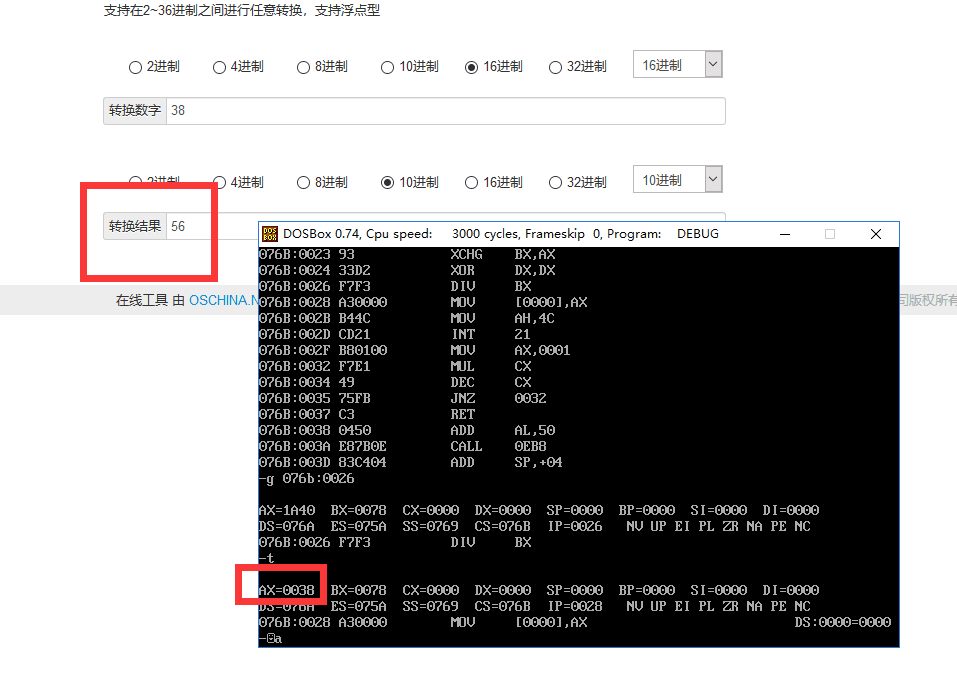• 设第ttt次迭代：计算dw[l]dw^{[l]}dw[l]、db[l]db^{[l]}db[l] on mini-batch。 Vdw=βVdw+(1−β)dwVdb=βVdb+(1−β)dbw=w−αVdwb=b−αVdb V_{dw}=\beta V_{dw}+(1-\beta)dw\\ V_{db}=\beta V_{db}+(1-\bet
梯度下降方法的计算公式（Momentum、RMSprop、Adam）Momentum梯度下降方法RMSprop梯度下降方法Adam梯度下降方法
Momentum梯度下降方法
设第$t$次迭代：计算$dw^{[l]}$、$db^{[l]}$ on mini-batch。
$V_{dw}=\beta V_{dw}+(1-\beta)dw\\ V_{db}=\beta V_{db}+(1-\beta)db\\ w=w-\alpha V_{dw}\\ b=b-\alpha V_{db}$
RMSprop梯度下降方法
设第$t$次迭代：计算$dw^{[l]}$、$db^{[l]}$ on mini-batch。
$S_{dw}=\beta S_{dw}+(1-\beta)dw^2\\ S_{db}=\beta S_{db}+(1-\beta)db^2\\ w=w-\alpha dw/(\sqrt {S_{dw}}+\epsilon)\\ b=b-\alpha db/(\sqrt {S_{db}}+\epsilon)$
Adam梯度下降方法
设第$t$次迭代：计算$dw^{[l]}$、$db^{[l]}$ on mini-batch。
$V_{dw}=\beta_{1} V_{dw}+(1-\beta_{1})dw\\ V_{db}=\beta_{1} V_{db}+(1-\beta_{1})db\\ S_{dw}=\beta_{2} S_{dw}+(1-\beta_{2})dw^2\\ S_{db}=\beta_{2} S_{db}+(1-\beta_{2})db^2\\ V^{corrected}_{dw}=V_{dw}/(1-\beta_{1}^t)\\ V^{corrected}_{db}=V_{db}/(1-\beta_{1}^t)\\ S^{corrected}_{dw}=S_{dw}/(1-\beta_{2}^t)\\ S^{corrected}_{db}=S_{db}/(1-\beta_{2}^t)\\ w=w-\alpha V^{corrected}_{dw}/(\sqrt {S^{corrected}_{dw}}+\epsilon)\\ b=b-\alpha V^{corrected}_{db}/(\sqrt {S^{corrected}_{db}}+\epsilon)$
其中，$\beta_{1}$=0.9、$\beta_{2}$=0.999、$\epsilon$=$10^{-8}$。


展开全文深度学习 算法
• 下列公式计算。 汇编代码，已调试 DATA SEGMENT TABLE DW L1,L2,L3,L4,L5,L6 MSG1 DB 'Please input an integer :$' MSG2 DB 'Y=$' MSG3 DB 'Illegally input!$' DATA ENDS STACK1 SEGMENT PARA STACK DW ... 问题描述： 从键盘上接收一位十进制数 X，计算 Y 值，并以十六进制形式显示出来，Y 按 下列公式计算。 汇编代码，已调试 DATA SEGMENT TABLE DW L1,L2,L3,L4,L5,L6 MSG1 DB 'Please input an integer :$'
MSG2 DB 'Y=$' MSG3 DB 'Illegally input!$'
DATA ENDS

STACK1 SEGMENT PARA STACK
DW 20H DUP(0)
STACK1 ENDS

CODE SEGMENT
ASSUME CS:CODE,DS:DATA,SS:STACK1
START:	MOV AX,DATA
MOV DS,AX

LEA DX,MSG1
MOV AH,09H
INT 21H		;输出提示信息

MOV AH,01H
INT 21H		;输入一个数字

CMP AL,33H
JB GEND
CMP AL,36H
JA GEND
AND AL,0FH	;转换为数字
XOR AH,AH	;高位清零
DEC AL		;获取x-1
SHL AL,1    	;计算L几
MOV BX,OFFSET TABLE ;获取table的内容
ADD BX,AX
JMP [BX]

GEND:
MOV DL,0AH
MOV AH,02H
INT 21H		;换行
LEA DX,MSG3
MOV	AH,09H
INT 21H		;显示提示错误信息
JMP L7

L1:		JMP GEND

L2: 	JMP GEND

L3:
CALL HHSC
MOV DL,31H
MOV AH,02H
INT 21H
MOV DL,32H
MOV AH,02H
INT 21H
JMP L7

L4:
CALL HHSC
MOV DL,38H
MOV AH,02H
INT 21H
JMP L7

L5:
CALL HHSC
MOV DL,32H
MOV AH,02H
INT 21H
MOV DL,35H
MOV AH,02H
INT 21H
JMP L7

L6:
CALL HHSC
MOV DL,31H
MOV AH,02H
INT 21H
MOV DL,38H
MOV AH,02H
INT 21H
JMP L7

L7:		MOV AH,4CH
INT 21H		;终止程序

HHSC PROC
MOV DL,0AH
MOV AH,02H
INT 21H		;换行
LEA DX,MSG2
MOV AH,09H
INT 21H		;显示输出信息
RET
HHSC ENDP

CODE ENDS

END START

结果展示展开全文• data segment m=8 n=3 res dw ?data endscode segment assume cs:code,ds:datastart: mov ax,data mov ds,ax mov cx,m call jc1;求m! mov bx,ax;bx=m! mov cx,n call...


data segment    m=8    n=3    res dw ?data endscode segment

assume cs:code,ds:datastart:    mov ax,data    mov ds,ax    mov cx,m    call jc1;求m!    mov bx,ax;bx=m!    mov cx,n    call jc1 ;求n!ax=n!    xchg ax,bx;要求m!/n!使用除法指令 div 由除法指令 应交换ax bx 此时ax=m! bx=n!    xor dx,dx    div bx;    mov bx,ax;bx=m!/n!    mov cx,m    sub cx,n    call jc1;ax=(m-n)!    xchg ax,bx;ax=m!/n! bx=(m-n)!    xor dx,dx;上一次做完除法之后可能会有余数保存在dx中 该程序不考虑余数，所以清零dx    div bx;ax=(m!/n!)/(m-n)!    mov res,ax    mov ah,4ch    int 21hjc1 proc;子程序 功能：求阶乘    mov ax,1L1: mul cx    dec cx    jnz L1    retjc1 endpcode endsend start赏

谢谢你请我吃糖支付宝微信


展开全文• 用数据窗口控件名.describe("Evaluate('计算公式',行号)") 理论上可以取到数据窗口里所有能用计算列计算的内容， 如： 1、取数据窗口的页数： dw_1.describe("Evaluate('pagecount()',1)") 2、取DropDownDW...

用数据窗口控件名.describe("Evaluate('计算公式',行号)") 理论上可以取到数据窗口里所有能用计算列计算的内容，

如：

1、取数据窗口的页数： dw_1.describe("Evaluate('pagecount()',1)")

2、取DropDownDW列显示的值： dw_1.describe("Evaluate('lookupdisplay(列名)',行号)")

3、取最小值： dw_1.describe("evaluate('min(billing_date_time)',1)")

4、求合计： dw_1.describe("evaluate('sum(charges for all)',1)")

说明：

Describe

可以描述DW中某个对象的某个属性的取值,虽然函数Describe可以获取对象的信息，但是表达式的取值就不能

正常读取了，而这又是经常遇到的。当行号为0时，表示应用于所有的行；如果表达式无效，返回结果为!

Evaluate

如果想获取数据窗口对象中由属性、函数等构成的表达式的取值时，必须在函数Describe中使用函数

Evaluate,LookUpDisplay

在数据窗口控件上显示的值和字段实际得到的值并不相同，使用函数GetItemX只能读取这样的字段的真实取值，而不是用户看到的值。如何才能读取用户看到的值？可以使用此函数,LookUpDisplay函数不能直接从PowerScript调用，可以在Describe中和Evaluate函数配合使用。因为函数Lookupdisplay不能指定对哪行数据进行操作，它的参数只有一个字段名称，所以必须和Evaluate函数配合使用。该函数的语法是：Lookupdisplay(columnname)参数columnname是字段的名称，而不是一个字符串。函数执行错误则返回空字符串。

例一：

ls_1   =   dw_1.Describe("dw_1.cb_4.text")

ls_2   =   This.Describe(dwo.name   +   ".ColType")

语法:   value   =   datawindow.Describe(string   ls)

例二：

判断第3行的sex是否为1，如果是则返回男，否则返回女dw_1.Describe("Evaluate('If(sex   =   1,   男,   女)   ',   3)")

语法:   Evalute('expression',rowno)其中，expression是属性表达式，rowno是要描述的行号。该函数放置在Describe的

属性列表中。

例三:

dw_1.Describe("Evaluate('Lookupdisplay(column)',"   +   string   (row   number)   +   ")")

在dw_1   里面有一个gxbm字段的edit属性页下面:dataWindow:d_dmzd_gxbm,Display   Column:dmmc,data

Column:gxbm.在显示时:不是显示gxbm的值,而是显示dmmc的值，但我们用getitemstring(row,"gxbm")时,得到的是gxbm的值，而不是显示的dmmc值,如果我们想得到显示的dmmc值,

那就用lookupdisplay来用:ls_1   =   dw_1.describe("Evaluate('lookupdisplay(gxbm)',"   +   string(1)   +   ")")

另：   在用代码学PB中有这样一段代码，值得关注：
integer   li_PageCount

//*******分页
li_PageCount   =   integer(dw_1.describe("evaluate('pagecount()',1)"))
i_int_currentpage   =   integer(dw_1.describe("evaluate('page()',1)"))
st_page.Text   =   "第"+String(   i_int_currentpage   )   +   "页（共"   +   String(   li_PageCount   )+"页）"

===========================================
//如何获取ddlb列表中的项(datavalue   和   displayvalue)

//参数：
//adw_1   数据窗口名
//columnname   当前列
//row   当前行
//ast_1   用作显示信息的静态文本控件

===========================================

DataWindowChild   Ldwc
String   Ls_CodeColumn
long   ll_row,ll_rowcount
String   ls_displaycolumn,ls_text   =   ' '
string   ls_prodinfo,   ls_prodname,   ls_prodnum
integer   li_tab,ll_item

if   adw_1.describe(Columnname+ '.edit.style ')= 'dddw '   then
adw_1.GetChild(Columnname,Ldwc)
Ls_CodeColumn=adw_1.DESCRIBE(Columnname+ '.DDDW.DATACOLUMN ')
ls_DisplayColumn =adw_1.DESCRIBE(Columnname+ '.DDDW.DisplayColumn ')
ldwc.setfilter( " ")
ldwc.filter()

if   ldwc.rowcount()> 10   then
ll_rowcount   =   10
else
ll_rowcount   =   ldwc.rowcount()
end   if

for   ll_row   =1   to   ll_rowcount
ls_text   =ls_text   +   ldwc.getitemstring(ll_row,Ls_CodeColumn)+ '- '+ldwc.getitemstring(ll_row,ls_DisplayColumn)+ '     '
next

ast_1.text   =   ls_text
elseif   adw_1.describe(Columnname+ '.edit.style ')= 'ddlb '   then
ls_prodinfo   = '0 '

ll_item   =   1
do   while   ls_prodinfo   <> ' '
ls_prodinfo   =   adw_1.GetValue(Columnname,   ll_item)

li_tab   =   Pos(ls_prodinfo,   "~t ",   1)

ls_prodname   =   Left(ls_prodinfo,   li_tab   -   1)

ls_prodnum   =   Mid(ls_prodinfo,   li_tab   +   1)

if   pos(ls_prodname, '－ ')   =0   then
ls_text   =   ls_text   +   ls_prodnum   + '－ '   +   ls_prodname+ '     '
else
ls_text   =   ls_text   +   ls_prodname+ '     '
end   if

ll_item   =   ll_item   +1
loop

ast_1.text   =   ls_text
else
ast_1.text   =   ' '

end   if

展开全文• 编写一个程序，计算 1 + 2 + 3 + 4 + … + 100 的累加和，并把累加和以 2 进制形式显示出来（要使用循环累加方法，不能使用公式 S=N*(N+1)/2=50*101=5050）。 DATA SEGMENT ;可以写入代码 DATA ENDS STACK SEGMENT...
• 我们知道离开Volume时候的光照亮度 Lo=Li*T+∫T*(Le(p,w)+σs(p,w)*∫p(w1,w)*L(w1)*dw1)*dt 其中T=exp(-∫(σs(p,w)+σa(p,w))*dt). ...我们注意到公式中的In-Scattering部分 ...第一次是计算四周的光线散...
• 一：数学原理当一幅二维数字图像从源图像N*M被放为(j*N) * (k*M)目标图像是，参照数学斜率计算公式必然有:(X1 – Xmin)/(Xmax - Xmin) = (Y1 - Ymin)/(Ymax-Ymin) 当Xmin 和 Ymin均为从零开始的像素点时，公式可以...
• z = (y - Xw )T (y - Xw) // y 列向量 X 矩阵 w 列向量 dz / dw = d{ ( y - Xw )T (y - Xw) } / dw dz / dw = d( tr{ ( y - ...// 由展开的计算公式而来，tr()为矩阵的迹 dz / dw = d( tr{ yTy - wTXTy - yTXw +...最小二乘 矩阵求导
• 一、logistic回归中的公式 二、向后计算偏导数 1.计算“da” 2.计算“dz” 使用链式求导法则 3.计算dw1”、“dw2” 4.更新w1、w2、b a这里是学习率
• 用数据窗口控件名.describe("Evaluate('计算公式',行号)") 理论上可以取到数据窗口里所有能用计算列计算的内容，如： 1、取数据窗口的页数：  dw_1.describe("Evaluate('pagecount()',1)") 2、取DropDownDW列...
• 最近在学习tensorflow时，发现在计算梯度时调用了一个函数GradientTape().gradient()函数。 以最简单的线性模型为例，y=W*x+b 根据上述公式可以计算dw，同理可以计算db。深度学习
• 在神经网络收敛的计算中，我们一直在使用梯度下降法，但除了梯度下降法，还有很多更加优秀的算法，可以适应...这样计算出来的v_dW不再只依赖于当前的dW,而是跟之前迭代结果的v_dW也有关系，通过变量β来调整过去与现...
• 上一篇定义了一个计算成本和梯度的正向传播和反向传播函数，计算出了cost和梯度dw，db 有了w，b和dw，db后需要更新一下w，b，继续算成本值 这一篇总结一下一次参数更新函数 w=w−adw(公式1)w=w-adw \tag{公式1}w=w−...深度学习 python 机器学习 算法 神经网络
• 1.RGB函数计算公式: 颜色值 ＝ (65536 * Blue) + (256 * Green) + (Red)  2.控件可拖动：  send(handle(this),274,61458,0)  3.如何用程序控制下拉子数据窗口的下拉和收起  用modify或者直接用dw_1.object....
• 1.RGB函数计算公式: 颜色值 ＝ (65536 * Blue) + (256 * Green) + (Red) 2.控件可拖动： send(handle(this),274,61458,0) 3.如何用程序控制下拉子数据窗口的下拉和收起 用modify或者直接用dw_1.object.col1....
• 1.RGB函数计算公式: 颜色值 ＝ (65536 * Blue) + (256 * Green) + (Red) 2.控件可拖动： send(handle(this),274,61458,0) 3.如何用程序控制下拉子数据窗口的下拉和收起 用modify或者直接用dw_1.object.col1....
• ## PB常用知识总结

千次阅读 2018-08-03 16:09:39
1.RGB函数计算公式:颜色值＝(65536 * Blue) + (256 * Green) + (Red) 2.控件可拖动： send(handle(this),274,61458,0) 3.如何用程序控制下拉子数据窗口的下拉和收起 用modify或者直接用dw_1.object.col1.dddw....
• ## PB技巧总结

千次阅读 2015-06-26 09:43:41
1.RGB函数计算公式: 颜色值 ＝ (65536 * Blue) + (256 * Green) + (Red) 2.控件可拖动：send(handle(this),274,61458,0) 3.如何用程序控制下拉子数据窗口的下拉和收起 用modify或者直接用dw_1.object.col1.dddw....
• 1.RGB函数计算公式: 颜色值 ＝ (65536 * Blue) + (256 * Green) + (Red) 2.控件可拖动： send(handle(this),274,61458,0) 3.如何用程序控制下拉子数据窗口的下拉和收起 用modify或者直接用dw_1.object.col1.dddw...
• 1.RGB函数计算公式: 颜色值 ＝ (65536 * Blue) + (256 * Green) + (Red)  2.控件可拖动： send(handle(this),274,61458,0) 3.如何用程序控制下拉子数据窗口的下拉和收起 用modify或者直接用dw_l1.dddw....
• ## PB常用技巧

千次阅读 2014-04-13 22:43:19
1.RGB函数计算公式: 颜色值＝ (65536 * Blue) + (256 * Green) + (Red) 2.控件可拖动： send(handle(this),274,61458,0) 3.如何用程序控制下拉子数据窗口的下拉和收起 用modify或者直接用dw_1.object.col1...
• 1.RGB函数计算公式: 颜色值 ＝ (65536 * Blue) + (256 * Green) + (Red)  2.控件可拖动：  send(handle(this),274,61458,0)  3.如何用程序控制下拉子数据窗口的下拉和收起  用modify或者直接用dw_1....